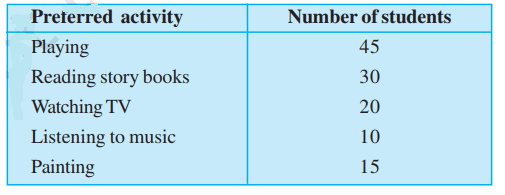# NCERT Class 6 Mathematics Ninth Chapter Data Handling Exercise 9.4 Solution

## EXERCISE 9.4

(1) A survey of 120 school students was done to find which activity they prefer to do in their free time.Draw a bar graph to illustrate the above data taking scale of 1 unit length = 5 students. Which activity is preferred by most of the students other than playing?(2) The number of Mathematics books sold by a shopkeeper on six consecutive days is shown below:Draw a bar graph to represent the above information choosing the scale of your choice.In case you are missed :- Previous Chapter Exercise Solution

(3) Following table shows the number of bicycles manufactured in a factory during the years 1998 to 2002.Illustrate this data using a bar graph. Choose a scale of your choice.(a) In which year was the maximum number of bicycles manufactured?

Ans: 2002

(b) In which year was the minimum number of bicycles manufactured?

Ans: 1999

(4) Number of persons in various age groups in a town is given in the following tableDraw a bar graph to represent the above information and answer the following questions. (take 1 unit length = 20 thousands)(a) Which two age groups have same population?

Ans: 30 – 44, 45 – 59

(b) All persons in the age group of 60 and above are called senior citizens. How many senior citizens are there in the town?

Ans: 1 lakh 20 thousand

In case you are missed :- Next Chapter Exercise Solution

Updated: April 14, 2023 — 3:06 pm